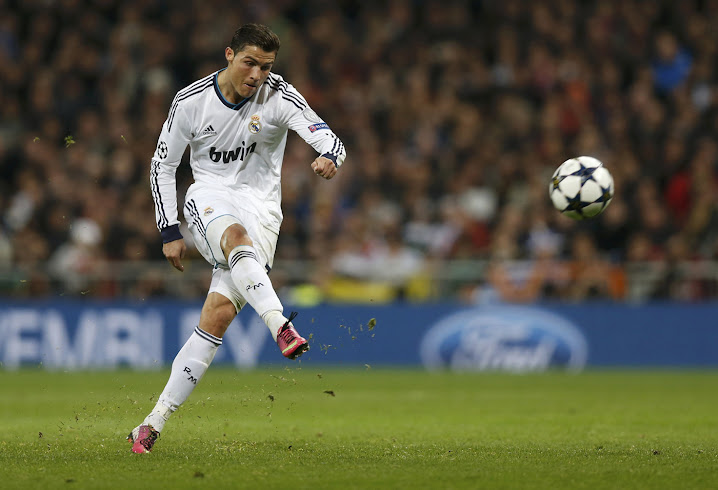Kinematics

While statics is concerned with bodies at rest or moving at a constant acceleration, dynamics is concerned with the accelerated motion of bodies. The study of dynamics developed much later than statics because of the need for accurate measurement of time. Galileo Galilee was a major early contributor, performing experiments with pendulums and falling bodies. Newton’s development of the three fundamental laws of motion was the springboard for increased understanding and work by other scientists. The two major branches of dynamics are kinematics, which is concerned with the geometric aspects of motion, and kinetics, which is concerned with the forces causing the motion.

In this lesson students will create a vehicle to learn important aspects of motion and freefall. Students will solve a problem that will help them to understand the kinematics concepts involved in projectile motion.

K1 – Describe freefall motion of a projectile as having constant velocity in the horizontal direction and uniformly accelerating motion in the vertical direction.

S1 – Calculate distance, displacement, speed, velocity, and acceleration from data.
S2 – Design, build, and test a machine that efficiently channels mechanical energy when friction and limited input energy are significant constraints.
S3 – Calculate acceleration due to gravity given data from a free-fall trajectory.
S4 – Calculate the x- and y-components of a projectile motion.
S5 – Determine the angle needed to launch a projectile a specific range given the projectile’s initial velocity.

Resources

Vandegrift High School | 9500 McNeil Drive, Austin, TX | T: 512 570 2300 |  Andrew.Perrone@leanderisd.org